# ISEE Middle Level Quantitative : Outcomes

## Example Questions

### Example Question #21 : Probability

Two standard decks of fifty-two cards, one with a red backing and one with a purple backing, are presented to you. The red deck has all of its aces removed; the purple deck has all of its kings removed. Which is the greater quantity?

(A) The probability that a card randomly drawn from the red deck is a seven

(B) The probability that a card randomly drawn from the purple deck is a seven

(A) is greater

It is impossible to determine which is greater from the information given

(A) and (B) are equal

(B) is greater

(A) and (B) are equal

Explanation:

Both modified decks still have four sevens, and both modified decks have forty-eight cards. Therefore, the probability of drawing a seven from the red deck is the same as the probability of drawing a seven from the purple deck: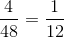.

### Example Question #21 : Data Analysis And Probability

The twos are removed from a standard fifty-two card deck and replaced with a king, a queen, a jack, and an ace from another deck. What is the probabliity that a card randomly drawn from this modified deck will be a club?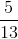It is impossible to answer the question from the information given.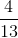It is impossible to answer the question from the information given.

Explanation:

The modified deck has had one card of each suit (including one club) removed from the deck, but has been replaced by four cards whose suits we do not know. There are anywhere from twelve to sixteen clubs in this modifiied deck, but without knowing exactly how many, we cannot answer this question.

### Example Question #22 : Probability

Billy has a bag of marbles with 5 red marbles, 6 green marbles and 7 blue marbles. What is the probability that Billy will choose a green marble when he reaches into the bag?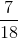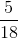Explanation:

Billy has a bag of marbles with 5 red marbles, 6 green marbles and 7 blue marbles. What is the probability that Billy will choose a green marble when he reaches into the bag?

In order to find the probability of anything we must divide the part by the whole.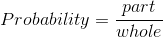We know that the part in this case are the green marbles; there are 6 of them.

To find the whole, we must add all the parts: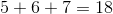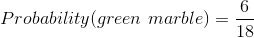We then reduce the fraction to get the final answer.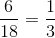### Example Question #22 : Data Analysis And Probability

Rosemary has a bag of marbles with 5 red marbles, 6 green marbles and 7 blue marbles. What is the probability that Rosemary will choose a blue marble when she reaches into the bag?Explanation:

Rosemary has a bag of marbles with 5 red marbles, 6 green marbles and 7 blue marbles. What is the probability that Rosemary will choose a blue marble when she reaches into the bag?

In order to find the probability of anything we must divide the part by the whole.We know that the part in this case are the blue marbles; there are 7 of them.

To find the whole, we must add all the parts: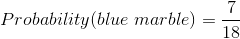### Example Question #25 : Data Analysis And Probability

The jacks are removed from a standard deck of 52 cards (Deck A) and added to another standard deck (Deck B). Which is the greater quantity?

(a) The probability that a card randomly drawn from Deck A will be a spade

(b) The probability that a card randomly drawn from Deck B will be a spade

(b) is the greater quantity

It is impossible to determine which is greater from the information given

(a) and (b) are equal

(a) is the greater quantity

(a) and (b) are equal

Explanation:

Removing the jacks from Deck A and putting them into Deck B transfers one spade and three other cards. The result is that:

12 out of 48 cards in Deck A will be spades - this is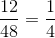of the cards; and,

14 out of 56 cards in Deck B will be spades - this is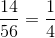of the cards.

This makes the probability of drawing a spade at random the same for both decks.

### Example Question #23 : Probability

The aces are removed from a standard deck of 53 cards (Deck A) and added to another standard deck of 53 (Deck B). Which is the greater quantity?

(a) The probability that a card randomly drawn from Deck A will be a heart

(b) The probability that a card randomly drawn from Deck B will be a heart

Note: Each deck includes the joker.

(a) and (b) are equal

(a) is the greater quantity

It is impossible to determine which is greater from the information given

(b) is the greater quantity

(b) is the greater quantity

Explanation:

Removing the aces from Deck A and putting them into Deck B transfers one heart and three other cards. The result is that:

12 out of 49 cards in Deck A will be hearts - this is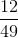of the cards; and,

14 out of 57 cards in Deck B will be hearts - this is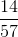of the cards.

To compare these probabilities, we express them in a common denominator as follows:

Deck A: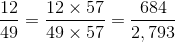Deck B: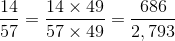The probability that a random draw from Deck B will yield a heart is slightly higher.

### Example Question #24 : Data Analysis And Probability

Catherine has a bag of marbles with 5 red marbles, 6 green marbles and 7 blue marbles. What is the probability that Catherine will choose a red marble when she reaches into the bag?Explanation:

Catherine has a bag of marbles with 5 red marbles, 6 green marbles and 7 blue marbles. What is the probability that Catherine will choose a red marble when she reaches into the bag?

In order to find the probability of anything we must divide the part by the whole.We know that the part in this case are the red marbles; there are 5 of them.

To find the whole, we must add all the parts: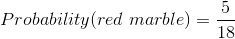### Example Question #22 : How To Find The Probability Of An Outcome

A large box contains some balls, each of which is marked with a number; one ball is marked with a "1", two balls are marked with a "2". and so forth up to ten balls with a "10".

Give the probability that a randomly drawn ball will be marked with a "5".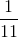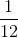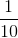Explanation:

The number of balls in the box, in total, will be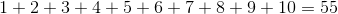balls,

5 of which will be marked with a "5".

The probability of drawing a "5" is therefore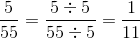### Example Question #21 : Probability

A large box contains some balls, each of which is marked with a number; one ball is marked with a "1", two balls are marked with a "2". and so forth up to ten balls with a "10".

A ball is drawn at random from this box. Which is the greater quantity?

(a) The probability that the ball will be marked with an even number

(b) The probability that the ball will be marked with an odd number

(b) is the greater quantity

(a) and (b) are equal

It is impossible to determine which is greater from the information given

(a) is the greater quantity

(a) is the greater quantity

Explanation:

The even numbers in the range of 1 to 10 are 2, 4, 6, 8, and 10; the number of balls marked with one of these numbers is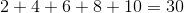Similarly, the number of balls marked with one of the odd numbers is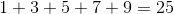There are more even-numbered balls than odd, so the probability of drawing an even-numbered ball is the greater.

### Example Question #21 : Probability

A pair of fair six-sided dice are thrown, and the sum of the numbers facing upward is noted. Which is the greater quantity?

(a) The probability that the sum will be even

(b)It is impossible to determine which is greater from the information given

(b) is the greater quantity

(a) is the greater quantity

(a) and (b) are equal

(a) and (b) are equal

Explanation:

There are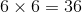possible rolls of two fair six-sided dice, each of which will come up with equal probability. The set of rolls is shown below, with the ways to roll an even number indicated.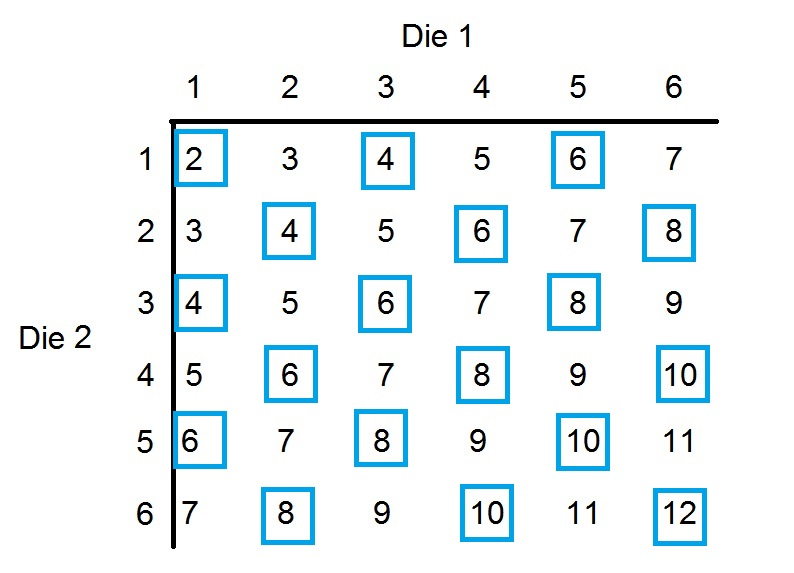There are 18 ways out of 36 to roll an even sum, making the probability of this event equal to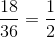.

### All ISEE Middle Level Quantitative Resources